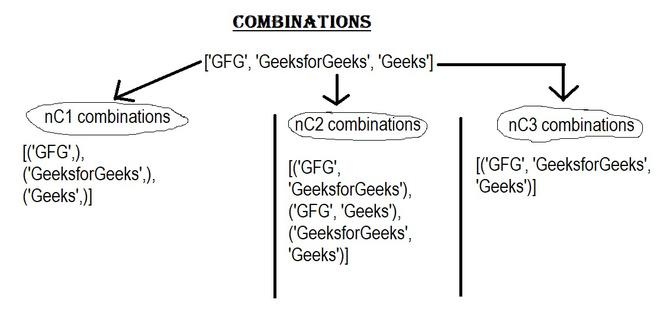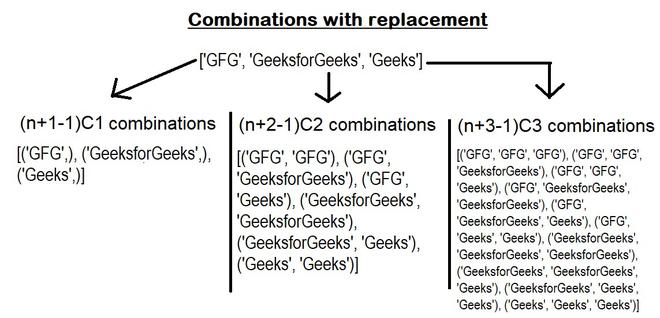Open in App
Not now

# All Combinations For A List Of Objects

• Difficulty Level : Easy
• Last Updated : 11 Dec, 2020

Prerequisite: Python Itertools

There are several ways to get all combinations for a list of objects in python. This problem has already a recursive solution. Python has an itertools module that provides two functions named combinations() and combinations_with_replacement() which make our work a lot easier. Below are the two methods:

#### 1. Using itertools.combinations():

Syntax : itertools.combination(iterable, r)

Where r is the length of output tuples.

This function returns subsequences(tuples) of length r from the input iterable. It takes a list of objects and the length of the output tuples(r) as input. But there are a few things to notice about this function like:

• The combination tuples are emitted in lexicographic order. So, if the input iterable is in sorted order the combined output will also be produced in sorted order.
• Elements are treated as unique based on their positions not on their values. So, if the input elements consist of duplicate values there will be duplicate values in the output.
• The number of items returned is nCr = n! / (r! * (n-r)!) when 0 <= r <= n; and zero when r > n.All combinations without replacement

Below is the implementation:

## Python3

 `# code ` `from` `itertools ``import` `combinations ` ` `  `# m = list of objects. ` `# same method can be applied  ` `# for list of integers. ` `m ``=` `[``'GFG'``, ``'GeeksforGeeks'``, ``'Geeks'``] ` `# display ` `for` `i ``in` `range``(``len``(m)): ` `  ``print``(``list``(combinations(m, i``+``1``))) `

Output:

```[('GFG',), ('GeeksforGeeks',), ('Geeks',)]
[('GFG', 'GeeksforGeeks'), ('GFG', 'Geeks'), ('GeeksforGeeks', 'Geeks')]
[('GFG', 'GeeksforGeeks', 'Geeks')]```

If the input has duplicate elements:

## Python3

 `# code ` `from` `itertools ``import` `combinations ` ` `  `# m = list of objects. ` `# 1st and 3rd elements are same.  ` `# same method can be applied  ` `# for list of integers. ` `m ``=` `[``'GFG'``, ``'GeeksforGeeks'``, ``'GFG'``] ` ` `  `# output : list of combinations. ` `for` `i ``in` `range``(``len``(m)): ` `  ``print``(``list``(combinations(m, i``+``1``))) `

Output:

```[('GFG',), ('GeeksforGeeks',), ('GFG',)]
[('GFG', 'GeeksforGeeks'), ('GFG', 'GFG'), ('GeeksforGeeks', 'GFG')]
[('GFG', 'GeeksforGeeks', 'GFG')]```

#### 2. Using itertools.combinations_with_replacement():

Syntax: itertools.combination_with_replacement(iterable, r)

Where r is the length of output tuples.

This function works same as itertools.combinations(). But this function returns r length subsequences including individual elements repeated more than once. There are also some points to note:

• The combination tuples are emitted in lexicographic order. So, if the input iterable is in sorted order the combined output will also be produced in sorted order.
• Elements are treated as unique based on their positions not on their values. So, if the input elements consist of duplicate values there will be duplicate values in the output.
• The number of items returned is (n+r-1)! / r! / (n-1)! when n > 0.Combinations with replacement.

Below is the implementation:

## Python3

 `# code ` `from` `itertools ``import` `combinations_with_replacement ` ` `  `# m = list of objects. ` `# same method can be applied  ` `# for list of integers. ` `m ``=` `[``'GFG'``, ``'GeeksforGeeks'``, ``'Geeks'``] ` ` `  `# output : list of combinations. ` `for` `i ``in` `range``(``len``(m)): ` `  ``print``(``list``(combinations_with_replacement(m, i``+``1``))) `

Output:

[(‘GFG’,), (‘GeeksforGeeks’,), (‘Geeks’,)]
[(‘GFG’, ‘GFG’), (‘GFG’, ‘GeeksforGeeks’), (‘GFG’, ‘Geeks’), (‘GeeksforGeeks’, ‘GeeksforGeeks’), (‘GeeksforGeeks’, ‘Geeks’), (‘Geeks’, ‘Geeks’)]
[(‘GFG’, ‘GFG’, ‘GFG’), (‘GFG’, ‘GFG’, ‘GeeksforGeeks’), (‘GFG’, ‘GFG’, ‘Geeks’), (‘GFG’, ‘GeeksforGeeks’, ‘GeeksforGeeks’), (‘GFG’, ‘GeeksforGeeks’, ‘Geeks’), (‘GFG’, ‘Geeks’, ‘Geeks’), (‘GeeksforGeeks’, ‘GeeksforGeeks’, ‘GeeksforGeeks’), (‘GeeksforGeeks’, ‘GeeksforGeeks’, ‘Geeks’), (‘GeeksforGeeks’, ‘Geeks’, ‘Geeks’), (‘Geeks’, ‘Geeks’, ‘Geeks’)]

My Personal Notes arrow_drop_up
Related Articles